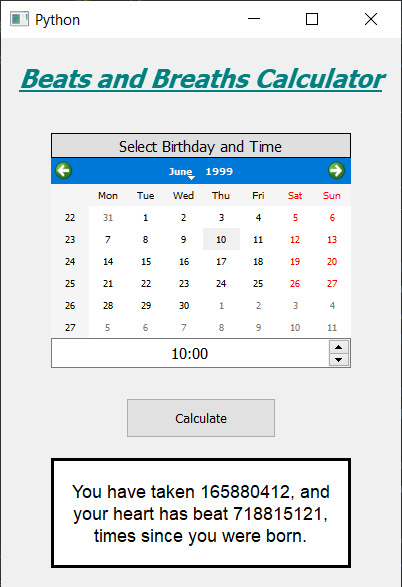# PyQt5 – Beats and Breaths Calculator

• Difficulty Level : Easy
• Last Updated : 16 Mar, 2022

In this article we will see how we can create a heart beats and breaths calculator using PyQt5. Below is how the calculator will look like.A normal resting heart rate for adults ranges from 60 to 100 beats per minute. Generally, a lower heart rate at rest implies more efficient heart function and better cardiovascular fitness. For example, a well-trained athlete might have a normal resting heart rate closer to 40 beats per minute.
Respiratory rate: A person’s respiratory rate is the number of breaths you take per minute. The normal respiration rate for an adult at rest is 12 to 20 breaths per minute. A respiration rate under 12 or over 25 breaths per minute while resting is considered abnormal.

GUI Implementation Steps :
1. Create a heading label that display the calculator name
2. Create label to show user to select the birth date and the birth time
3. Create a QCalendarWidget object for user to select the birth date
4. Create a QTimeEdit object to get the birth time
5. Create a push button to calculate the heart beats and breaths
6. Create a label to show the calculated beats and breaths
Back-End Implementation :
1. Make the calendar future date block i.e set current date as maximum date
2. Add action to the push button
3. Inside the push button action get the date from the calendar and time from the QTimeEdit
4. Get the day, month and year from the date and hour and minute from the time edit
5. Create a datetime object for current date and the birth date
6. Get the difference from both the dates and get the days and seconds
7. Convert days and seconds into minutes
8. Get the heart beats and breaths by multiplying minutes with the average rates.
9. Show the calculated beats and breaths with the help of label

Below is the implementation

## Python3

 `# importing libraries``from` `PyQt5.QtWidgets ``import` `*``from` `PyQt5 ``import` `QtCore, QtGui``from` `PyQt5.QtGui ``import` `*``from` `PyQt5.QtCore ``import` `*``import` `datetime``import` `sys`  `class` `Window(QMainWindow):` `    ``def` `__init__(``self``):``        ``super``().__init__()` `        ``# setting title``        ``self``.setWindowTitle("Python ")` `        ``# width of window``        ``self``.w_width ``=` `400` `        ``# height of window``        ``self``.w_height ``=` `550` `        ``# setting geometry``        ``self``.setGeometry(``100``, ``100``, ``self``.w_width, ``self``.w_height)` `        ``# calling method``        ``self``.UiComponents()` `        ``# showing all the widgets``        ``self``.show()` `    ``# method for components``    ``def` `UiComponents(``self``):``        ``# creating head label``        ``head ``=` `QLabel("Beats ``and` `Breaths Calculator", ``self``)` `        ``head.setWordWrap(``True``)` `        ``# setting geometry to the head``        ``head.setGeometry(``0``, ``10``, ``400``, ``60``)` `        ``# font``        ``font ``=` `QFont(``'Times'``, ``15``)``        ``font.setBold(``True``)``        ``font.setItalic(``True``)``        ``font.setUnderline(``True``)` `        ``# setting font to the head``        ``head.setFont(font)` `        ``# setting alignment of the head``        ``head.setAlignment(Qt.AlignCenter)` `        ``# setting color effect to the head``        ``color ``=` `QGraphicsColorizeEffect(``self``)``        ``color.setColor(Qt.darkCyan)``        ``head.setGraphicsEffect(color)` `        ``# creating a label``        ``b_label ``=` `QLabel("Select Birthday ``and` `Time", ``self``)` `        ``# setting properties  label``        ``b_label.setAlignment(Qt.AlignCenter)``        ``b_label.setGeometry(``50``, ``95``, ``300``, ``25``)``        ``b_label.setStyleSheet("QLabel"``                              ``"{"``                              ``"border : ``1px` `solid black;"``                              ``"background : rgba(``70``, ``70``, ``70``, ``25``);"``                              ``"}")``        ``b_label.setFont(QFont(``'Times'``, ``9``))` `        ``# creating a calendar widget to select the date``        ``self``.calendar ``=` `QCalendarWidget(``self``)` `        ``# setting geometry of the calendar``        ``self``.calendar.setGeometry(``50``, ``120``, ``300``, ``180``)` `        ``# setting font to the calendar``        ``self``.calendar.setFont(QFont(``'Times'``, ``6``))` `        ``# getting current date``        ``date ``=` `QDate.currentDate()` `        ``# blocking future dates``        ``self``.calendar.setMaximumDate(date)` `        ``# creating a time edit object to receive time``        ``self``.time ``=` `QTimeEdit(``self``)` `        ``# setting properties to the time``        ``self``.time.setGeometry(``50``, ``300``, ``300``, ``30``)``        ``self``.time.setAlignment(Qt.AlignCenter)``        ``self``.time.setFont(QFont(``'Times'``, ``9``))` `        ``# creating a push button``        ``calculate ``=` `QPushButton("Calculate", ``self``)` `        ``# setting geometry to the push button``        ``calculate.setGeometry(``125``, ``360``, ``150``, ``40``)` `        ``# adding action to the calculate button``        ``calculate.clicked.connect(``self``.calculate_action)` `        ``# creating a label to show percentile``        ``self``.result ``=` `QLabel(``self``)` `        ``# setting properties to result label``        ``self``.result.setAlignment(Qt.AlignCenter)``        ``self``.result.setGeometry(``50``, ``420``, ``300``, ``110``)``        ``self``.result.setWordWrap(``True``)``        ``self``.result.setStyleSheet("QLabel"``                                  ``"{"``                                  ``"border : ``3px` `solid black;"``                                  ``"background : white;"``                                  ``"}")``        ``self``.result.setFont(QFont(``'Arial'``, ``11``))` `    ``def` `calculate_action(``self``):``        ``# getting birth date day``        ``birth ``=` `self``.calendar.selectedDate()` `        ``# getting year and month day of birth day``        ``birth_year ``=` `birth.year()``        ``birth_month ``=` `birth.month()``        ``birth_day ``=` `birth.day()` `        ``# getting time of from the time edit``        ``time ``=` `self``.time.time()` `        ``# getting hour and seconds``        ``hour ``=` `time.hour()``        ``minute ``=` `time.minute()` `        ``# getting today date``        ``current ``=` `QDate.currentDate()` `        ``# getting year and month day of current day``        ``current ``=` `datetime.datetime.now()` `        ``# converting  date into date object``        ``birth_date ``=` `datetime.datetime(birth_year, birth_month, birth_day, hour, minute, ``0``)` `        ``# getting difference in both the dates``        ``difference ``=` `current ``-` `birth_date` `        ``# getting difference in days``        ``days ``=` `difference.days` `        ``# setting seconds``        ``seconds ``=` `difference.seconds` `        ``# converting days and seconds into minutes``        ``minutes ``=` `seconds ``/` `60` `+` `days ``*` `24` `*` `60` `        ``heartbeat ``=` `int``(minutes ``*` `65``)` `        ``breath ``=` `int``(minutes ``*` `15``)` `        ``# setting this value with the help of label``        ``self``.result.setText("You have taken " ``+` `str``(breath) ``+``                            ``", ``and` `your heart has beat " ``+` `str``(heartbeat) ``+``                            ``" times since you were born.")`  `# create pyqt5 app``App ``=` `QApplication(sys.argv)` `# create the instance of our Window``window ``=` `Window()` `# start the app``sys.exit(App.``exec``())`

Output :

My Personal Notes arrow_drop_up